Actually, we've already been working with quadratics. They are the trinomial guys we've been factoring: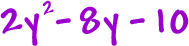What makes them quadratics is that they are 2nd degree polynomials.

 (They have a squared guy like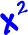or.)

Now, we're going to be solving equations with them like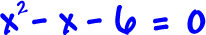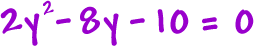Here's the big official form of a quadratic equation:Examples: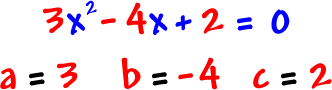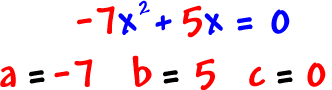It would be silly to ever have a = 0 in a quadratic -- Then we wouldn't have a squared guy!